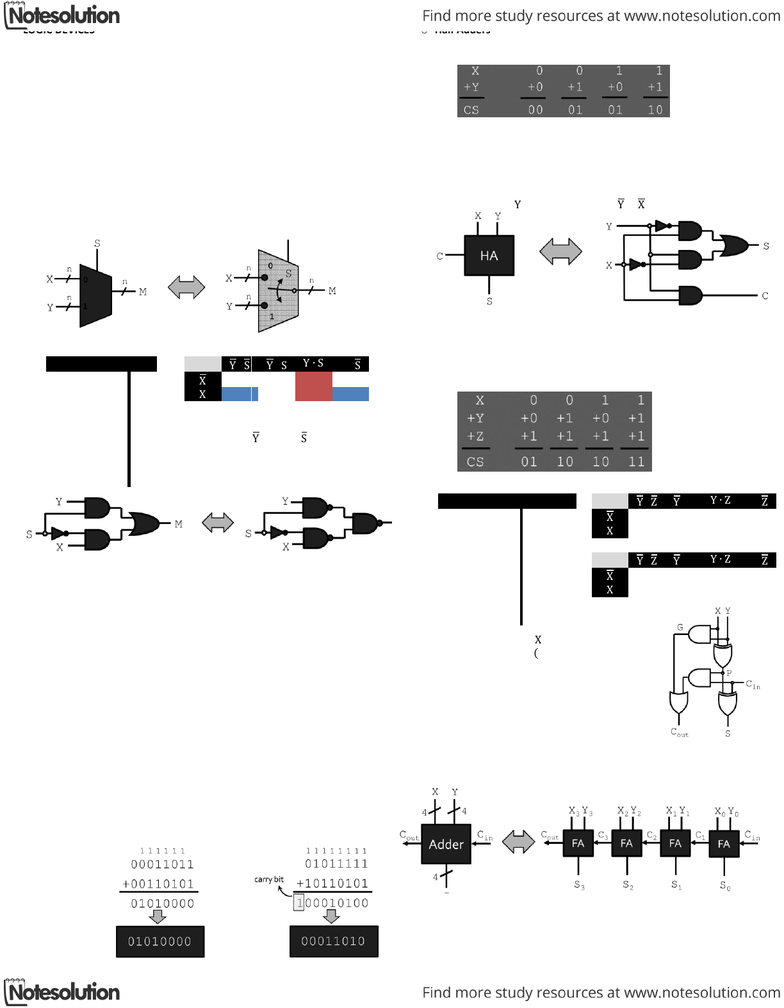# CSC258H1 Lecture Notes - Multiplexer, Binary Number

34 views1 pages
School
UTSG
Department
Computer Science
Course
CSC258H1
Professor
Page:
of 1LOGIC DEVICES
Building up from gates
o Some common and more complex structures
Multiplexers (MUX)
Subtractors
Comparators
Decoders
Seven-segment decoders
o Certain structures are common to many circuits, and have block
elements of their own
Karnaught map review moved to jan18ce
Multiplexers (MUX)
o Behavior:
output is X if S = 0; otherwise output is Y if S = 1
o Multiplexer design
X
Y
S
M
M
 
0
0
0
0
0
0
1
0
0
0
1
0
1
0
1
1
0
1
0
0
0
1
1
1
1
0
0
1
    
1
0
1
0
1
1
0
1
1
1
1
1
o Also known as binary adders
Small circuits devices that add two digits together
Combind together to create interative combinational circuits
Like an OXR for sum (s) and AND for carry (c)
o Binary Math review
Each digit of a demical number represents a power of 10:
258 = 2*102 + 5*101 x+ 8*100
Each digit of a binary number represents a power of 2:
011012 = 0*24 + 1*23 + 1*22 + 0*21 + 1*20
= 1310
27 + 53 95 + 181
27 = 00011011 95 = 01011111
53 = 00110101 181 = 10110101
A 2-input, 1-bit width binary adder that performs the following
computations:
The sum is expressed as a sum bit S and a carry bit C
Equations and circuits for half adder units are easy to
define (even w/o k-maps)
      
Similar to half-adders, but with another input Z, which
represents a carry-in bit
C and Z are sometimes labels as Cout and Cin
When Z is 0, the unit behaves exactly like a half adder
When Z is 1, the full adder performs the following computations
X
Y
S
C
C
 
 
0
0
0
0
0
0
1
0
0
0
1
0
0
1
1
1
0
1
0
0
0
1
1
1
S
 
 
1
0
0
0
0
1
0
1
1
0
1
1
1
0
1
0
1
1
0
1
1
1
1
1
         
      
  
Then let carry generate  
And let carry propagate   
Resultant circuit